# Python Pandas Series

• Last Updated : 16 Mar, 2023

Pandas Series is a one-dimensional labeled array capable of holding data of any type (integer, string, float, python objects, etc.).

## Python3

```
# import pandas as pd
import pandas as pd

# simple array
data = [1, 2, 3, 4]

ser = pd.Series(data)
print(ser)

```

Output :

```0    1
1    2
2    3
3    4
dtype: int64
```

The axis labels are collectively called index. Pandas Series is nothing but a column in an excel sheet.
Labels need not be unique but must be a hashable type. The object supports both integer and label-based indexing and provides a host of methods for performing operations involving the index.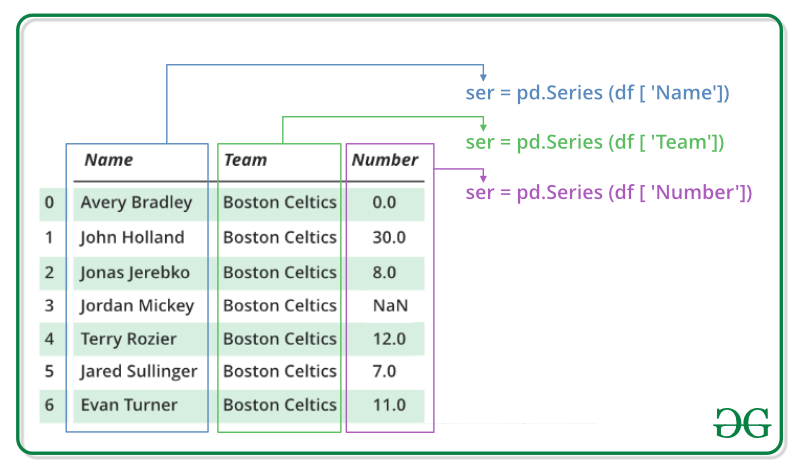## Python Pandas Series

We will get a brief insight on all these basic operations which can be performed on Pandas Series :

## Creating a Pandas Series

In the real world, a Pandas Series will be created by loading the datasets from existing storage, storage can be SQL Database, CSV file, and Excel file. Pandas Series can be created from the lists, dictionary, and from a scalar value etc. Series can be created in different ways, here are some ways by which we create a series:

Creating a series from array: In order to create a series from array, we have to import a numpy module and have to use array() function.

## Python3

```
# import pandas as pd
import pandas as pd

# import numpy as np
import numpy as np

# simple array
data = np.array(['g','e','e','k','s'])

ser = pd.Series(data)
print(ser)

```

Output :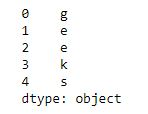Creating a series from Lists:
In order to create a series from list, we have to first create a list after that we can create a series from list.

```
import pandas as pd

# a simple list
list = ['g', 'e', 'e', 'k', 's']

# create series form a list
ser = pd.Series(list)
print(ser)

```

Output :For more details refer to Creating a Pandas Series

## Accessing element of Series

There are two ways through which we can access element of series, they are :

• Accessing Element from Series with Position
• Accessing Element Using Label (index)

Accessing Element from Series with Position : In order to access the series element refers to the index number. Use the index operator [ ] to access an element in a series. The index must be an integer. In order to access multiple elements from a series, we use Slice operation.

Accessing first 5 elements of Series

```
# import pandas and numpy
import pandas as pd
import numpy as np

# creating simple array
data = np.array(['g','e','e','k','s','f', 'o','r','g','e','e','k','s'])
ser = pd.Series(data)

#retrieve the first element
print(ser[:5])

```

Output :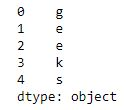Accessing Element Using Label (index) :
In order to access an element from series, we have to set values by index label. A Series is like a fixed-size dictionary in that you can get and set values by index label.

Accessing a single element using index label

```
# import pandas and numpy
import pandas as pd
import numpy as np

# creating simple array
data = np.array(['g','e','e','k','s','f', 'o','r','g','e','e','k','s'])
ser = pd.Series(data,index=[10,11,12,13,14,15,16,17,18,19,20,21,22])

# accessing a element using index element
print(ser)

```

Output :

```o
```

For more details refer to Accessing element of Series

## Indexing and Selecting Data in Series

Indexing in pandas means simply selecting particular data from a Series. Indexing could mean selecting all the data, some of the data from particular columns. Indexing can also be known as Subset Selection.

Indexing a Series using indexing operator `[]` :
Indexing operator is used to refer to the square brackets following an object. The `.loc` and `.iloc` indexers also use the indexing operator to make selections. In this indexing operator to refer to df[ ].

```# importing pandas module
import pandas as pd

# making data frame

ser = pd.Series(df['Name'])
data
```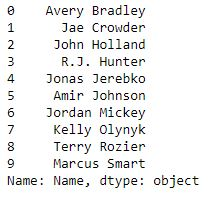Now we access the element of series using index operator [ ].

```# using indexing operator
data[3:6]
```

Output :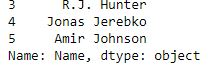Indexing a Series using `.loc[ ]` :
This function selects data by refering the explicit index . The `df.loc` indexer selects data in a different way than just the indexing operator. It can select subsets of data.

```# importing pandas module
import pandas as pd

# making data frame

ser = pd.Series(df['Name'])
data
```Now we access the element of series using `.loc[]` function.

```# using .loc[] function
data.loc[3:6]
```

Output :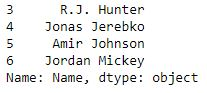Indexing a Series using `.iloc[ ]` :
This function allows us to retrieve data by position. In order to do that, we’ll need to specify the positions of the data that we want. The `df.iloc `indexer is very similar to `df.loc `but only uses integer locations to make its selections.

```# importing pandas module
import pandas as pd

# making data frame

ser = pd.Series(df['Name'])
data
```Now we access the element of Series using `.iloc[]` function.

```# using .iloc[] function
data.iloc[3:6]
```

Output :## Binary Operation on Series

We can perform binary operation on series like addition, subtraction and many other operation. In order to perform binary operation on series we have to use some function like `.add()`,`.sub()` etc..
Code #1:

```# importing pandas module
import pandas as pd

# creating a series
data = pd.Series([5, 2, 3,7], index=['a', 'b', 'c', 'd'])

# creating a series
data1 = pd.Series([1, 6, 4, 9], index=['a', 'b', 'd', 'e'])

print(data, "\n\n", data1)
```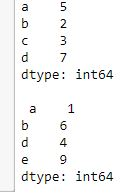Now we add two series using `.add()` function.

```# adding two series using
```

Output :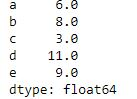Code #2:

```# importing pandas module
import pandas as pd

# creating a series
data = pd.Series([5, 2, 3,7], index=['a', 'b', 'c', 'd'])

# creating a series
data1 = pd.Series([1, 6, 4, 9], index=['a', 'b', 'd', 'e'])

print(data, "\n\n", data1)
```Now we subtract two series using `.sub` function.

```# subtracting two series using
# .sub
data.sub(data1, fill_value=0)
```

Output :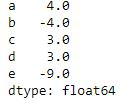For more details refer to Binary operation methods on series

## Conversion Operation on Series

In conversion operation we perform various operation like changing datatype of series, changing a series to list etc. In order to perform conversion operation we have various function which help in conversion like `.astype()`, `.tolist()` etc.
Code #1:

```# Python program using astype
# to convert a datatype of series

# importing pandas module
import pandas as pd

# reading csv file from url

# dropping null value columns to avoid errors
data.dropna(inplace = True)

# storing dtype before converting
before = data.dtypes

# converting dtypes using astype
data["Salary"]= data["Salary"].astype(int)
data["Number"]= data["Number"].astype(str)

# storing dtype after converting
after = data.dtypes

# printing to compare
print("BEFORE CONVERSION\n", before, "\n")
print("AFTER CONVERSION\n", after, "\n")
```

Output :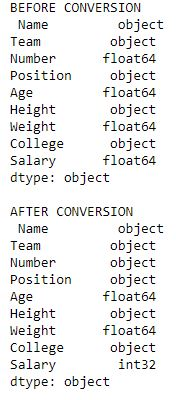Code #2:

```# Python program converting
# a series into list

# importing pandas module
import pandas as pd

# importing regex module
import re

# making data frame

# removing null values to avoid errors
data.dropna(inplace = True)

# storing dtype before operation
dtype_before = type(data["Salary"])

# converting to list
salary_list = data["Salary"].tolist()

# storing dtype after operation
dtype_after = type(salary_list)

# printing dtype
print("Data type before converting = {}\nData type after converting = {}"
.format(dtype_before, dtype_after))

# displaying list
salary_list

```

Output :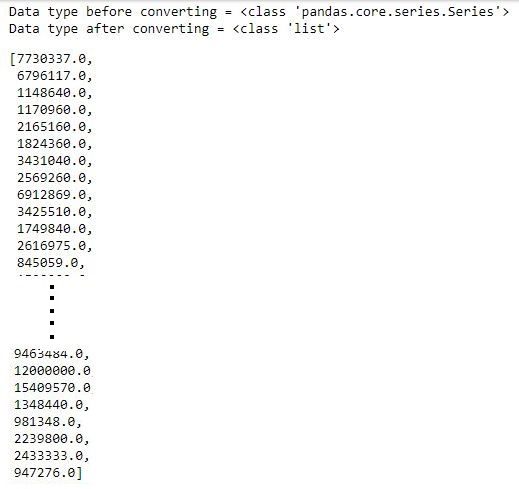Binary operation methods on series:

Function Description
add() Method is used to add series or list like objects with same length to the caller series
sub() Method is used to subtract series or list like objects with same length from the caller series
mul() Method is used to multiply series or list like objects with same length with the caller series
div() Method is used to divide series or list like objects with same length by the caller series
sum() Returns the sum of the values for the requested axis
prod() Returns the product of the values for the requested axis
mean() Returns the mean of the values for the requested axis
pow() Method is used to put each element of passed series as exponential power of caller series and returned the results
abs() Method is used to get the absolute numeric value of each element in Series/DataFrame
cov() Method is used to find covariance of two series

Pandas series method:

Function Description
Series() A pandas Series can be created with the Series() constructor method. This constructor method accepts a variety of inputs
combine_first() Method is used to combine two series into one
count() Returns number of non-NA/null observations in the Series
size() Returns the number of elements in the underlying data
name() Method allows to give a name to a Series object, i.e. to the column
is_unique() Method returns boolean if values in the object are unique
idxmax() Method to extract the index positions of the highest values in a Series
idxmin() Method to extract the index positions of the lowest values in a Series
sort_values() Method is called on a Series to sort the values in ascending or descending order
sort_index() Method is called on a pandas Series to sort it by the index instead of its values
head() Method is used to return a specified number of rows from the beginning of a Series. The method returns a brand new Series
tail() Method is used to return a specified number of rows from the end of a Series. The method returns a brand new Series
le() Used to compare every element of Caller series with passed series.It returns True for every element which is Less than or Equal to the element in passed series
ne() Used to compare every element of Caller series with passed series. It returns True for every element which is Not Equal to the element in passed series
ge() Used to compare every element of Caller series with passed series. It returns True for every element which is Greater than or Equal to the element in passed series
eq() Used to compare every element of Caller series with passed series. It returns True for every element which is Equal to the element in passed series
gt() Used to compare two series and return Boolean value for every respective element
lt() Used to compare two series and return Boolean value for every respective element
clip() Used to clip value below and above to passed Least and Max value
clip_lower() Used to clip values below a passed least value
clip_upper() Used to clip values above a passed maximum value
astype() Method is used to change data type of a series
tolist() Method is used to convert a series to list
get() Method is called on a Series to extract values from a Series. This is alternative syntax to the traditional bracket syntax
unique() Pandas unique() is used to see the unique values in a particular column
nunique() Pandas nunique() is used to get a count of unique values
value_counts() Method to count the number of the times each unique value occurs in a Series
factorize() Method helps to get the numeric representation of an array by identifying distinct values
map() Method to tie together the values from one object to another
between() Pandas between() method is used on series to check which values lie between first and second argument
apply() Method is called and feeded a Python function as an argument to use the function on every Series value. This method is helpful for executing custom operations that are not included in pandas or numpy

My Personal Notes arrow_drop_up